# Sine Bar: Working Principle, Function, Formula, Applications & Limitations

The sine bar is most commonly used to measure the angles of different metallic parts. This article explains the sine bar formula, working principle, construction, and applications.

## What is a Sine Bar?

A sine bar is a measuring tool used to determine the unknown angles of metallic components or workpieces. It is made up of two precision cylinders connected at each end of a hard-ground body.

There is a precisely controlled distance between the centers of two cylinders or rollers. The top of the bar is parallel to the central line between the two cylinders.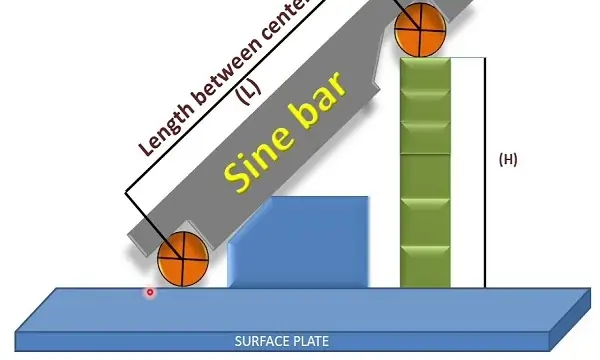To prevent tear and wear while handling and to make it strong, high-chromium, high-carbon corrosion resistance steel is used in the manufacturing of the sine bar.

An entire number is used as the dimension between the two rollers to make it easier for the observer, and it makes the hypotenuse of a triangle when used.

## Working Principle of the Sine Bar

A dial gauge and a gauge block are both used with the sine bar when measuring the angles of a workpiece. The main goal of the measurement here is to make a surface where the dial gauge is placed horizontally.

The working principle of the sine bar is based on the law of trigonometry. For example, if a block is located on a horizontal bench and you want to measure the angle of the block, you need to place the bar on the inclined surface of the block.

After the placement of the sine bar on the block, your bar will be tilted exactly like the block. Here, the gauge blocks are used to make the top surface horizontally.

The ratio of the height of the gauge blocks (H) and the distance between the centers of the rollers (L) is equal to the sine of the angle of inclination (sin(Ɵ)) of the block.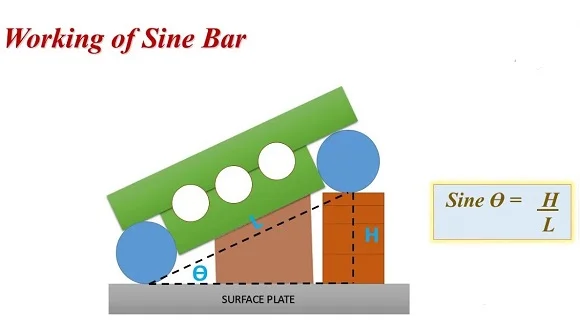When the sine bar is placed on any leveled surface, the top edge of the bar and the surface will be parallel. But if you raise any of the rollers using gauge blocks, the top edge of the bar will tilt at some angle with respect to that surface. Hence, this tilted bar will form an angle that can be measured using the sine rule.

• Hypotenuse is constant (10 inches or 100 mm).
• The sine rule is used to measure the angle. Incrementing at 1-minute intervals yields angles ranging from 0 to 90 degrees. Sine (angle) = opposite/hypotenuse
• Height is measured from the distance between the table surface and the bottom of one roller.
• This tool is used to set or measure the angles.

## Sine Bar Construction

• The gauge body of the bar is made up of a rigid material like steel. Each end of this bar has a roller. Both rollers connected at the end of the bar have the same diameter and have parallel axes.
• The central line between the rollers and the steel bar’s top is parallel.
• The distance between the two rollers should be the same as the length of the sine bar.
• The bar’s length is very precise and can be either 100 mm, 200 mm, or 300 mm.
• There are relief holes to lessen the bus sine bar weight.

This bar alone can’t precisely measure the component’s angles. Elevation gauges and Slip gauges are always needed with the sine bar to measure the angles of any component.

## Components of Sine Bar

The sine bar has the following components:

• Surface plate
• Vernier height gauge
• Slip gauge or block gauge
• Dial gauge

#### 1) Surface Plate

The sine bar contains a surface plate which is utilized as a base on which the bar, height gauges, and slip gauges are arranged. The main objective of the surface plate is to provide a particular horizontal surface to the sine bar.

If you place the bar on the surface plate, then both the bar and the horizontal surface of the surface plate must be parallel.

#### 2) Vernier Height Gauge

The sine bar roller’s height is measured by using the vernier height gauge. It is then used to measure the angle of the large workpiece.

#### 3) Block Gauge or Slip Gauge

In the sine bar arrangement, the length or height of the workpiece is measured precisely using a slip gauge or block gauge.

#### 4) Dial Gauge

Surface uniformity is checked through a dial gauge. A surface is considered parallel to the dial gauge base if it shows no deflection when moving on the surface.

When placing sine bars, the dial gauge uses to see if the top surface of the component is parallel to the surface plate. Or you can say it is used to calculate the angle of the tapered sine of the component that has to be measured.

## Working of Sine Bar

A sine bar can be used in various ways to measure the angle of various kinds of components. The following are two various working methods of the sine bar:

• Working when measuring the angle of a large workpiece
• Working when measuring the angle of a small workpiece or part

### 1) Working when measuring the angle of a small Workpiece

When measuring the angle of the small workpiece, you need to place the roller of the bar at some specific position between the sine bar and the surface plate.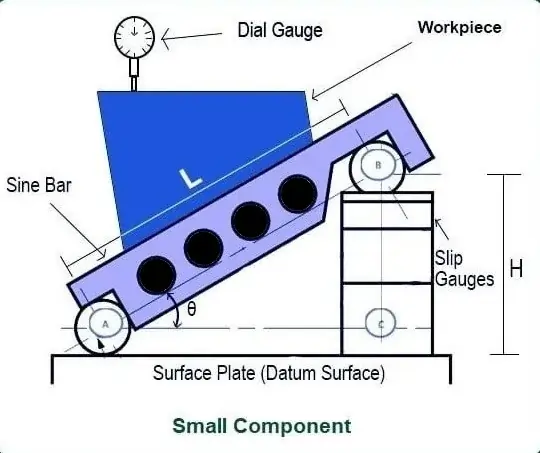• The workpiece to be measured is placed on top of the sine bar. With the help of the dial gauges, the top of the workpiece is checked to see if it corresponds to the surface plate or not.
• The dial gauge travels over the workpiece throughout its length.
• The deflection of the dial gauge shows that the surface plate and the surface of the workpiece are not precisely parallel.
• The height of the slip gauge can now be adjusted by removing or adding slip gauge blocks.
• This height is adjusted until the dial gauge shows a zero reading on the entire length of the workpiece.
• Once the dial gauge shows zero, the angle of the workpiece now becomes the same as the angle of this bar above the surface plate.
• At that point, the sine bar’s angle (Ɵ) is equal to the sine inverse to the height H to length L ratio.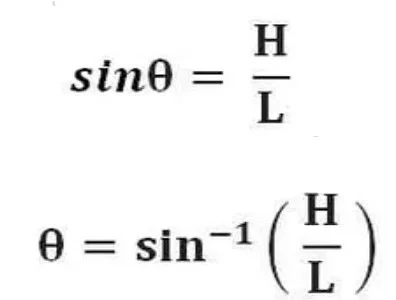Here:

• L is the length of the bar
• H is the height of the slip gauges
• ϴ is the angle of the workpiece or component

### 2) Working while measuring the angle of a large Workpiece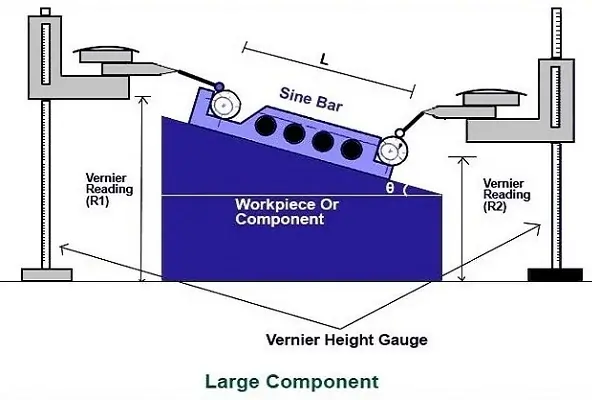• While measuring the big or large block or workpiece, the bar is installed above the block, as it will not be possible to place the workpiece on this bar.
• In this condition, the bottom of the block should be parallel to the other surfaces. On keeping the bar above the block, the top side of the block will be bent, and we have to measure its angle.
• Here the bar will be in an inclined position, and hence the height of both of the rollers will be different.
• Measure the heights of the rollers with the help of the vernier height gauge. The heights of both of these rollers will be noted as H1 and H2, and H1 is greater than H2.
• Here, H1–H2 is the rise of the sine bar. A dial gauge helps in measuring the measuring pressure.

Here is the formula for the calculation of the angle of the large components: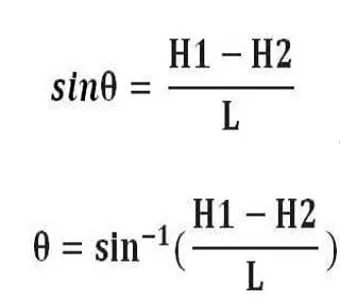Here:

• H2 is the height of the lower cylinder
• H1 is the height of the upper cylinder
• Ɵ is the component’s angle
• L is the length of the sine bar

## Applications of Sine Bar

• To set the block or workpiece at the required angles.
• To check the unknown angles of the block.
• Some types of these bars need the workpiece to be mounted in order to run the conical-shaped machining on the workpiece.
• It is also used to measure unknown angles on some heavy components.
• Taper-key angles can also be checked with the help of a sine bar.
• It precisely checks the flatness of any surface.

Read More: Heat Pump Working and Types

## Limitations of Sine Bar

• Wrong measurements are recorded in the case of an unknown projection in the workpiece.
• The construction of slip gauges is complicated and lacks a proper building approach. A trial-and-error procedure should be considered while building it, and that is why it requires a lot of time.
• The bar should always be greater in length than the workpiece.
• If the workpiece is lengthy, getting such a long sine bar will be impossible. As a solution, the sine bar and the height gauges are used together for the measurement.

### What is a sine table used for?

Sine plates and sine tables are used to check the compound or simple angular relationship of one diameter to another with high accuracy. It is also utilized to create accurate angular diameters or faces in simple and compound planes through light, job boring, or grinding machining.

### What are the types of sine bars?

1. Sine Center: It measures the angles of the conical workpieces that have male and female parts. This type of sine bar can’t calculate an angle of more than 45 degrees.
2. Sine Table: This wide and large sine bar locks itself after positioning. That’s why it can hold the workpiece during the whole measuring process.
3. Compound Sine Table: This type of sine bar is used for large components, which you can’t measure with the simple bar. In the compound sine table, two sine tables are installed at the right angle and can be rotated as per measurement requirements.

### What is the sine bar used for?

A sine bar is used to determine the unknown angles of metallic components or workpieces. It is made up of two precision cylinders connected at each end of a hard-ground body.